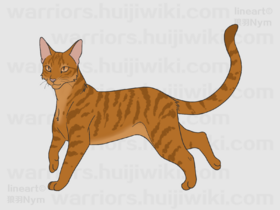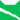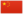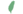—— 火心，关于蕨毛，《险路惊魂》，第94页

Brackenfur雷族 武士蕨崽非官 Brackenkit  小蕨非官
 蕨爪 Brackenpaw  蕨掌
 蕨毛 Brackenfur  蕨毛

Q2353：雷族公猫，自《呼唤野性》登场的角色

## 细节

### 外貌

• 他是一只琥珀色眼睛:271的金棕色虎斑公猫，:猫物表口鼻泛灰，:286四肢修长，:214身侧上有一道疤痕。:54

### 作者声明

• 维琪认为他和刺掌不是同卵双胞胎。
• 维琪乐见狮心成为他和刺掌的父亲，但矛盾的是，她也曾提到狮心没有孩子。

### 趣闻

• 他和姐妹炭毛成为学徒时都未满六个月。:93

### 勘误

• 他曾被错误地描述为姜黄色、:95:146淡姜色、:165暗姜色、:38浅棕色:92:108和铁锈色。:92
• 在《武士之心》中，他曾被错误地描绘为为白色的口鼻、尾尖和脚爪。:11不过，全彩版修正了此错误。
• 他曾被错误地描述为绿色眼睛。:75
• 他曾被错误地描述为母猫。:287
• 尽管他已在《疑云重重》中获得了武士名号，但在后来的《虎掌的愤怒》中他仍被错误地称为学徒。:2章
• 他曾在担任武士时被错误地称呼为长老:144
• 黑莓掌曾被错误地称呼为他。:184

## 登场书目

• 呼唤野性 (Q57)[配角][首次登场🐱]
• 寒冰烈火 (Q59)[配角]
• 疑云重重 (Q60)[配角]
• 风起云涌 (Q65)[配角]
• 险路惊魂 (Q66)[配角]
• 力挽狂澜 (Q67)[配角]
• 午夜追踪 (Q139)[配角]
• 新月危机 (Q140)[配角]
• 重现家园 (Q141)[配角]
• 星光指路 (Q142)[配角]
• 黄昏战争 (Q143)[配角]
• 日落和平 (Q144)[配角]
• 预视力量 (Q152)[配角]
• 暗河汹涌 (Q153)[配角]
• 驱逐之战 (Q154)[配角]
• 天蚀遮月 (Q155)[配角]
• 暗夜长影 (Q156)[配角]
• 拂晓之光 (Q157)[配角]
• 第四学徒 (Q158)[配角]
• 战声渐近 (Q159)[配角]
• 暗夜密语 (Q160)[配角]
• 月光印记 (Q161)[配角]
• 武士归来 (Q162)[配角]
• 群星之战 (Q163)[配角]
• 学徒探索 (Q170)[配角]
• 雷影交加 (Q171)[配角]
• 天空破碎 (Q172)[猫物闲角]
• 极夜无光 (Q173)[配角]
• 烈焰焚河 (Q174)[配角]
• 风暴来袭 (Q175)[配角]
• 迷失群星 (Q3563)[配角]
• 静默冰融 (Q3579)[配角]
• 叠影重障 (Q3624)[对话提及]
• 暗由心生 (Q4183)[配角]
• 无星之地 (Q4385)[猫物闲角]
• 迷雾明光 (Q4621)[猫物闲角]
• 河翻浪涌 (Q4643)[配角]
• 天穹将倾 (Q4799)[对话提及]
• 火星的探索 (Q176)[配角]
• 黑莓星的风暴 (Q182)[配角]
• 虎心的阴影 (Q185)[猫物闲角]
• 鸦羽的拷问 (Q186)[猫物闲角]
• 松鼠飞的希冀 (Q3561)[配角]
• 灰条的誓言 (Q4057)[配角]
• 一星的告解 (Q4826)[配角]
• 冬青叶的故事 (Q187)[猫物闲角]
• 雾星的征兆 (Q188)[猫物闲角]
• 虎掌的愤怒 (Q190)[对话提及]
• 叶池的祈愿 (Q191)[配角]
• 鸽翅的沉默 (Q192)[配角]
• 红尾的恩债 (Q3565)[配角]
• 褐皮的族群 (Q3566)[猫物闲角]
• 蛾翅的秘密 (Q3628)[猫物闲角]
• 黛西的至亲 (Q3629)[猫物闲角]
• 斑毛的反抗 (Q4337)[猫物闲角]
• 黑脚的审判 (Q4336)[猫物闲角][仅于回忆]
• 变革之风 (Q4411)[配角]
• 逐出影族 (Q4769)[配角]
• 武士之心 (Q215)[配角]
• 族群的秘密 (Q199)[追溯修订]
• 族群的战争 (Q202)[配角]
• 终极指南 (Q204)[配角]

## 参考文献

这篇文章基于CC BY-SA 3.0许可使用了猫武士维基（英语）Brackenfur一文中的部分内容。
1. 《寒冰烈火》第八章 (Q776)
2. 呼唤野性 (Q57)
3. 迷失群星 (Q3563)
4. 某时间点 — 《寒冰烈火》第八章 (Q776)
5. Kate (2016-03-06). 站内截图. BlogClan . 原页面 归档于 2018-04-04.
6. 《黑脚的审判》第六章
7. 《寒冰烈火》第八章
8. 午夜追踪 (Q139)预视力量 (Q152)
9. 月光印记
10. 寒冰烈火
11. 黑莓星的风暴
12. 午夜追踪
13. 预视力量
14. Vicky Holmes (2016-04-21). 站内截图. Facebook . 原页面 归档于 2016-04-21. “No, I don't think so. As far as I know, this is very very rare among cats.”
15. Victoria Holmes (2009-09-12). 站内截图. Facebook . 原页面 归档于 2017-11-01. “Though I love the idea of Lionheart fathering Thornclaw and Brackenfur - Brackenfur is totally like lovely Lion!”
16. Victoria Holmes (2009-12-01). 站内截图. Facebook . 原页面 归档于 2017-11-01. “Just checking my notes from BP... Nope, don't think he did, sorry.”
17. 力挽狂澜
18. 风起云涌
19. 疑云重重
20. 暗夜密语
21. 武士之心
22. 乌爪的旅程
23. 武士归来
24. 黄昏战争
25. 虎掌的愤怒
26. 雷族神偷